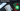# Hackerrank | Solution of Drawing Book in Golang

April 7th, 2020
|

In this one we will solve question Drawing Book from hackerrank in golang. Let's dive right into it.

# Problem Statement

The question can be found at this link.

The problem statement states that we are given a book with `n` pages. We need to turn to page `p`, what is the minimum number of page turns required to reach page `p` given certain constraints

# Constraints and Challenges

The problem lists the following constraints

• Pages can be turned from front or back
• The front always starts with Page `1` on the right page
• The back can have printed pages on both sides or one side depending on the number of pages

# Solution

The only challenge here is to compute the number of page turns it will take from front and back. After that, it's just about finding which is less. There are three cases here.

• Turning pages from front
• Turning pages from back when total pages are odd
• Turning pages from back when total pages are even

Let's look at all these individually.

## Turning pages from front

The first page always starts at `1`. So if we need to turn to page `1`, we need `zero` turns, for page `2`, `1` turn, for page `3`, `1` turn, for page `4`, `2` turns, for page `5`, `2` turns and so on...If we notice, if we divide page by `2`, we get the number of turns.

## Turning pages from back when total pages are odd and even

If you form the same series on a piece of paper, you will conclude that for odd pages the turns is equal to `(n-p)/2` and for even `(n+1-p)/2`

Once this is done, it's just about finding which is less

We have discussed the approach, I urge you to go ahead on hackerrank and give it another try.If you are here, it means something went wrong in implementation or you are just too lazy. In any case, let's see a simple implementation of the above logic.
``````package main

import "fmt"

func main() {
var n, p int
fmt.Scan(&n, &p)
front := p / 2

back := (n - p) / 2

if n%2 == 0 {
back = (n + 1 - p) / 2
}

if front < back {
fmt.Println(front)
} else {
fmt.Println(back)
}
}``````

Nothing very fancy in the code. We just converted our discussion into code.

# Summary

So, we solved the drawing book problem from hackerrank.

I hope you enjoyed solving this question. This is it for this one, complete source code for this post can be found on my Github Repo. Will see you in the next one.